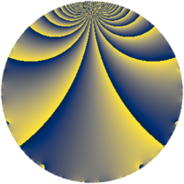# Properties

 Label 845.2.cLevel $845$ Weight $2$ Character orbit 845.c Rep. character $\chi_{845}(506,\cdot)$ Character field $\Q$ Dimension $50$ Newform subspaces $8$ Sturm bound $182$ Trace bound $4$

# Related objects

## Defining parameters

 Level: $$N$$ $$=$$ $$845 = 5 \cdot 13^{2}$$ Weight: $$k$$ $$=$$ $$2$$ Character orbit: $$[\chi]$$ $$=$$ 845.c (of order $$2$$ and degree $$1$$) Character conductor: $$\operatorname{cond}(\chi)$$ $$=$$ $$13$$ Character field: $$\Q$$ Newform subspaces: $$8$$ Sturm bound: $$182$$ Trace bound: $$4$$ Distinguishing $$T_p$$: $$2$$

## Dimensions

The following table gives the dimensions of various subspaces of $$M_{2}(845, [\chi])$$.

Total New Old
Modular forms 106 50 56
Cusp forms 78 50 28
Eisenstein series 28 0 28

## Trace form

 $$50q + 4q^{3} - 46q^{4} + 50q^{9} + O(q^{10})$$ $$50q + 4q^{3} - 46q^{4} + 50q^{9} - 2q^{10} - 12q^{14} + 46q^{16} - 4q^{17} + 20q^{22} - 16q^{23} - 50q^{25} + 28q^{27} - 20q^{30} + 4q^{35} - 2q^{36} + 44q^{38} + 6q^{40} - 64q^{42} - 24q^{43} - 56q^{48} - 50q^{49} - 4q^{51} - 24q^{53} + 8q^{55} + 80q^{56} - 4q^{61} + 8q^{62} - 74q^{64} - 52q^{66} + 64q^{68} + 44q^{69} - 40q^{74} - 4q^{75} - 20q^{77} - 36q^{79} + 18q^{81} + 48q^{82} - 4q^{87} - 48q^{88} + 42q^{90} - 12q^{92} - 4q^{94} - 16q^{95} + O(q^{100})$$

## Decomposition of $$S_{2}^{\mathrm{new}}(845, [\chi])$$ into newform subspaces

Label Dim. $$A$$ Field CM Traces $q$-expansion
$$a_2$$ $$a_3$$ $$a_5$$ $$a_7$$
845.2.c.a $$2$$ $$6.747$$ $$\Q(\sqrt{-1})$$ None $$0$$ $$-4$$ $$0$$ $$0$$ $$q+iq^{2}-2q^{3}+q^{4}+iq^{5}-2iq^{6}+\cdots$$
845.2.c.b $$4$$ $$6.747$$ $$\Q(\zeta_{8})$$ None $$0$$ $$0$$ $$0$$ $$0$$ $$q+(\zeta_{8}+\zeta_{8}^{2})q^{2}-\zeta_{8}^{3}q^{3}+(-1-2\zeta_{8}^{3})q^{4}+\cdots$$
845.2.c.c $$4$$ $$6.747$$ $$\Q(i, \sqrt{5})$$ None $$0$$ $$0$$ $$0$$ $$0$$ $$q+\beta _{1}q^{2}+(1+2\beta _{2})q^{3}+(1+\beta _{2})q^{4}+\cdots$$
845.2.c.d $$4$$ $$6.747$$ $$\Q(i, \sqrt{13})$$ None $$0$$ $$4$$ $$0$$ $$0$$ $$q+\beta _{1}q^{2}+q^{3}+(-2+\beta _{3})q^{4}-\beta _{2}q^{5}+\cdots$$
845.2.c.e $$4$$ $$6.747$$ $$\Q(\zeta_{12})$$ None $$0$$ $$4$$ $$0$$ $$0$$ $$q-\zeta_{12}^{2}q^{2}+(1-\zeta_{12}^{3})q^{3}-q^{4}+\zeta_{12}q^{5}+\cdots$$
845.2.c.f $$6$$ $$6.747$$ 6.0.153664.1 None $$0$$ $$-10$$ $$0$$ $$0$$ $$q+\beta _{1}q^{2}+(-1-\beta _{2}-\beta _{4})q^{3}+\beta _{2}q^{4}+\cdots$$
845.2.c.g $$8$$ $$6.747$$ 8.0.22581504.2 None $$0$$ $$-4$$ $$0$$ $$0$$ $$q+\beta _{1}q^{2}+(-1-\beta _{3})q^{3}+(-1+\beta _{2}+\cdots)q^{4}+\cdots$$
845.2.c.h $$18$$ $$6.747$$ $$\mathbb{Q}[x]/(x^{18} + \cdots)$$ None $$0$$ $$14$$ $$0$$ $$0$$ $$q+(-\beta _{1}+\beta _{6})q^{2}+(1+\beta _{11})q^{3}+(-2+\cdots)q^{4}+\cdots$$

## Decomposition of $$S_{2}^{\mathrm{old}}(845, [\chi])$$ into lower level spaces

$$S_{2}^{\mathrm{old}}(845, [\chi]) \cong$$ $$S_{2}^{\mathrm{new}}(65, [\chi])$$$$^{\oplus 2}$$$$\oplus$$$$S_{2}^{\mathrm{new}}(169, [\chi])$$$$^{\oplus 2}$$